# Two-phase flow in porous media: Analytical vs numerical solution

We've been trying to use the analytical (Buckley-Leverett) solution of the two-phase flow in porous media to fit the Corey-type relative permeability model to the experimental oil recovery data. In this post, I'm going to compare the numerical solution of the same model with the analytical results. You can find the codes that I have written in this github repository. Here, I only call the codes and compare the results.

In :
# load the input parameters and the functions
using Roots, PyPlot, Dierckx, JFVM
import JSON, JLD
include("../../../projects/peteng/rel_perms.jl")
include("../../../projects/peteng/forced_imbibition_corey.jl")
include("../../../projects/peteng/frac_flow_funcs.jl")
IJulia.clear_output()


The input parameters are stored in the input_params_BL.jld file, that can be loaded by

In :
JLD.@load "../../../projects/peteng/input_params_BL.jld";


Now we can run the functions for the analytical and numerical solutions:

In :
# call the functions
# numerical solution (finite volume)
(t_num, R_num, sw_prf)=forced_imb_impes(mu_water, mu_oil, u_inj,
poros, perm_ave, swc, sor, kro0, no,
krw0,nw, swi, 1.0, L_core, pv_inj, Nx=50)

# Analytical solution (BL)
(xt_shock, sw_shock, xt_prf, sw_prf, t_anal, p_inj, R_anal) = frac_flow_wf(
muw=mu_water, muo=mu_oil, ut=u_inj, phi=poros,
k=perm_ave, swc=swc, sor=sor, kro0=kro0, no=no,
krw0=krw0, nw=nw, sw0=swi, sw_inj=1.0, L=L_core, pv_inj=pv_inj)
IJulia.clear_output() # only to clear the output from the previous function


Now, we can plot the results and compare the solutions:

In :
plot(t_anal, R_anal, "o", t_num, R_num)
xlabel("time [s]")
ylabel("Recovery factor [-]")
IJulia.clear_output()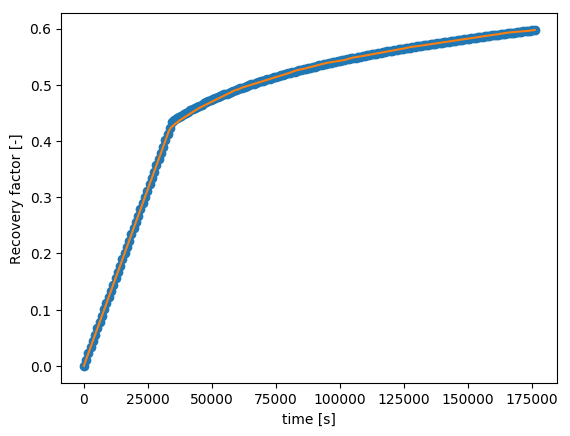It seems that the match very well. But if we zoom on the recovery plot close to the water breakthrough time,

In :
plot(t_anal, R_anal, "o", t_num, R_num)
xlabel("time [s]")
ylabel("Recovery factor [-]")
axis([25000, 50000, 0.40, 0.5])
IJulia.clear_output()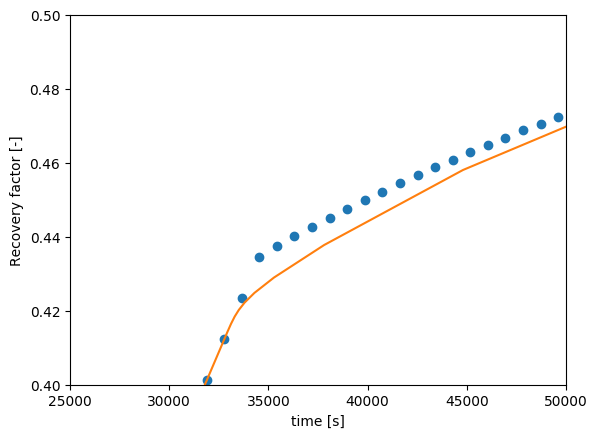we can see that there is roughly 1 percent underestimation of the recovery factor by the numerical method. One reason is the numerical diffusion in the upwind scheme that I have used in my numerical solution. With this diffusion, the front is not sharp anymore and the water breakthrough (decrease in the slope of the recovery curve from the linear trend) happens a bit earlier in time. Let's test it by plotting the saturation profiles:

In :
pv_inj2 = 0.3
(t_num, R_num, sw_prf_num)=forced_imb_impes(mu_water, mu_oil, u_inj,
poros, perm_ave, swc, sor, kro0, no,
krw0,nw, swi, 1.0, L_core, pv_inj2, Nx=50)

# Analytical solution (BL)
(xt_shock, sw_shock, xt_prf, sw_prf, t_anal, p_inj, R_anal) = frac_flow_wf(
muw=mu_water, muo=mu_oil, ut=u_inj, phi=poros,
k=perm_ave, swc=swc, sor=sor, kro0=kro0, no=no,
krw0=krw0, nw=nw, sw0=swi, sw_inj=1.0, L=L_core, pv_inj=pv_inj2)
visualizeCells(sw_prf_num)
plot(xt_prf*t_anal[end], sw_prf)
axis([0, L_core, 0, 1.0])
legend(["Numerical", "Analytical"])
xlabel("Core length [m]")
ylabel("Water saturation [-]")
IJulia.clear_output() # only to clear the output from the previous function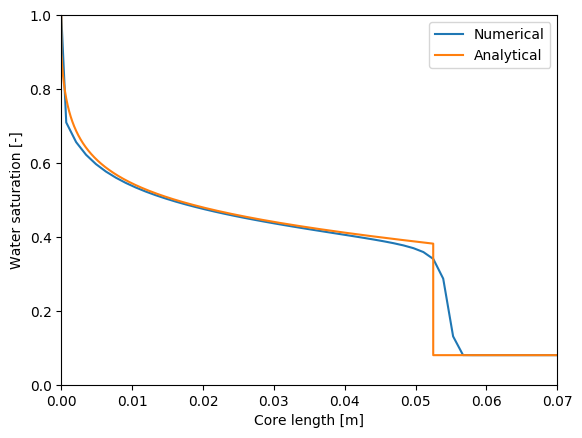We can clearly see that the extra numerical diffusion causes the water front to move faster resulting in an earlier water breakthrough. We can decrease this diffusion by refining the grid:

In :
(t_num, R_num, sw_prf_num)=forced_imb_impes(mu_water, mu_oil, u_inj,
poros, perm_ave, swc, sor, kro0, no,
krw0,nw, swi, 1.0, L_core, pv_inj2, Nx=500)
visualizeCells(sw_prf_num)
plot(xt_prf*t_anal[end], sw_prf)
axis([0, L_core, 0, 1.0])
legend(["Numerical", "Analytical"])
xlabel("Core length [m]")
ylabel("Water saturation [-]")
IJulia.clear_output()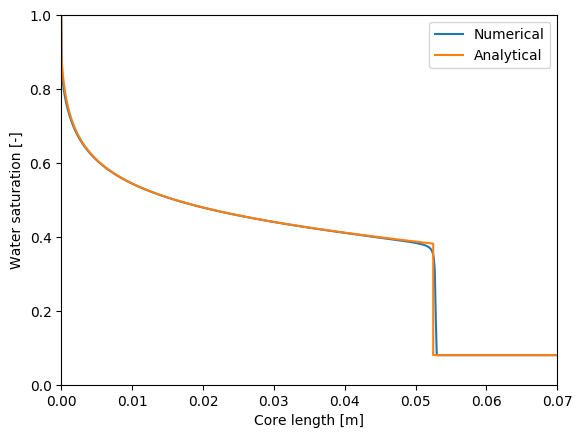Now, we can see that the numerical solution is very close to the analytical solution. This must give a better match for the recovery curves as well:

In :
# numerical solution (finite volume)
(t_num, R_num, sw_prf)=forced_imb_impes(mu_water, mu_oil, u_inj,
poros, perm_ave, swc, sor, kro0, no,
krw0,nw, swi, 1.0, L_core, pv_inj, Nx=500)

# Analytical solution (BL)
(xt_shock, sw_shock, xt_prf, sw_prf, t_anal, p_inj, R_anal) = frac_flow_wf(
muw=mu_water, muo=mu_oil, ut=u_inj, phi=poros,
k=perm_ave, swc=swc, sor=sor, kro0=kro0, no=no,
krw0=krw0, nw=nw, sw0=swi, sw_inj=1.0, L=L_core, pv_inj=pv_inj)
plot(t_anal, R_anal, "o", t_num, R_num)
xlabel("time [s]")
ylabel("Recovery factor [-]")
axis([25000, 50000, 0.40, 0.5])
IJulia.clear_output()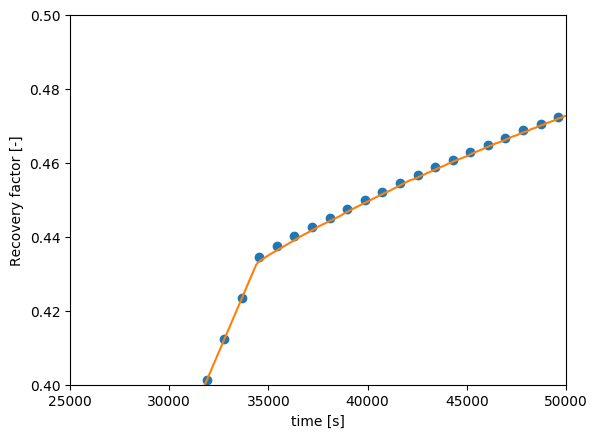# Heat transport in porous media

In this post, I will solve the energy balance equation for the flow of a single phase fluid in porous media. I don't use my work time to write in this blog. But this is an exception. The code is going to be used by one of our students and it is a good idea to document it here.

### The physical system¶

We have a L = 100 m x W = 20 m rectangular domain, that represent a cross section of a reservoir at 2000 m depth (i.e., 200 bar initial reserveoir pressure) with a porosity of 35% and a permeability of 2 mDarcy, saturated with brine at 80 degrees Celsius. Cold water at 25 degrees Celsius is injected with a Darcy velocity of 1 m/day from the mid section of the left side of the domain, which in turn pushes brine out of the domain from the right side (mid section). We assume that the top and bottom boundaries are closed to flow. But the temperature is fixed at 80 degrees Celsius. We know that the density and viscosity of water changes with temperature, therefore we need a relation for them, which we can find by using the CoolProp.jl package. Let's create a table of values:

In :
# You can install CoolProp by:
# Pkg.build("CoolProp")
using CoolProp, DataFrames, PyPlot, Polynomials, JFVM
# a table of density values
p_res = 200e5        # [Pa]
T_res = 80 + 273.15 # [K]
T_inj = 25 + 273.15 # [K]
T     = linspace(T_inj, T_res, 10)
rho_water = zeros(length(T))
mu_water  = zeros(length(T))
for i in eachindex(T)
rho_water[i] = PropsSI("D", "T", T[i], "P", p_res, "water")           # [kg/m^3]
mu_water[i]  = PropsSI("viscosity", "T", T[i], "P", p_res, "water")   # [Pa.s]
end
# display as a beautiful table with DataFrames
DataFrame(T_K = round(T, 2), μ_Pa_s = round(mu_water,6), ρ_kg_per_m3 = round(rho_water,2))

WARNING: Method definition describe(AbstractArray) in module StatsBase at /home/ali/.julia/v0.5/StatsBase/src/scalarstats.jl:573 overwritten in module DataFrames at /home/ali/.julia/v0.5/DataFrames/src/abstractdataframe/abstractdataframe.jl:407.

Out:
T_K μ_Pa_s ρ_kg_per_m3
1 298.15 0.000888 1005.84
2 304.26 0.000779 1003.98
3 310.37 0.00069 1001.83
4 316.48 0.000617 999.43
5 322.59 0.000556 996.78
6 328.71 0.000504 993.92
7 334.82 0.000459 990.85
8 340.93 0.000421 987.58
9 347.04 0.000388 984.13
10 353.15 0.000359 980.49

It is possible to fit a polynomial function to the above table of data, using the Polynomials.jl package

In :
mu_fit  = polyfit(T, mu_water, 2)
rho_fit = polyfit(T, rho_water, 2)
subplot(2,1,1)
scatter(T, mu_water, label = "μ data")
plot(T, polyval(mu_fit, T))
xlabel("T [K]")
ylabel("Viscosity [Pa.s]")
legend()
axis([minimum(T), maximum(T), minimum(mu_water), maximum(mu_water)])

subplot(2,1,2)
scatter(T, rho_water, label = "ρ data")
plot(T, polyval(rho_fit, T))
xlabel("T [K]")
ylabel("Density [kg/m^3]")
legend();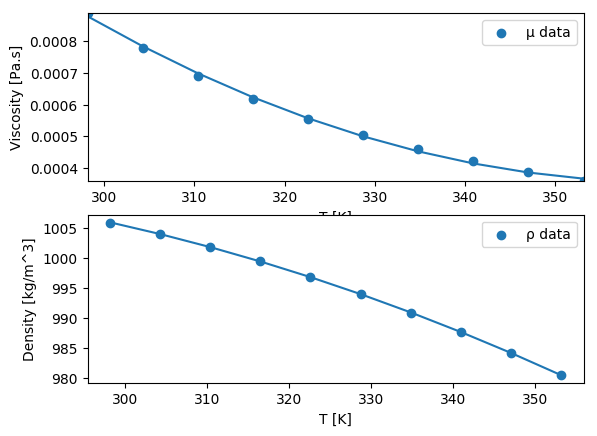The above fit looks fine. Now we can go into the fun PDE part. The PDE's that we are going to solve read: $$\frac{\partial}{\partial t}\left(\varphi\rho\right)+\nabla.\left(\rho\mathbf{u}\right)=0,$$ $$\mathbf{u}=-\frac{k}{\mu}\nabla p,$$ $$\begin{multline*} \frac{\partial}{\partial t}\left(\varphi\rho c_{p,w}\left(T-T_{0}\right)+\left(1-\varphi\right)\rho_{s}c_{p,s}\left(T-T_{0}\right)\right)+\\ +\nabla.\left(-\lambda\nabla T+\rho c_{p,w}\left(T-T_{0}\right)\mathbf{u}\right)=0 \end{multline*}$$

I'm not going to the details of the above equations, and I hope there is no mistakes since I wrote them from memory. The only change that I made here is that I replace $T-T_0$ with a new variable $\theta$. The discretization is done as usual. You can see that the PDE's are coupled. However, I do not use a fully coupled solution. All I do is that I calculate the values of viscosity and density of water (that are temperature-dependent) from the previous time-step, find the pressure profile, then velocity field, and finally a new temperature profile by solving the energy equation. I loop through this a couple of times and update the result in each time-step.
Let's define the domain and all the physical parameters.

### Physical parameters¶

In :
T0    = 25.0 + 273.15  # [K] reference temperature
L     = 100            # [m]
W     = 20             # [m]
poros = 0.35           # [-]
perm  = 0.002e-12      # Darcy
V_dp  = 0.2            # Dykstra-Parsons coef
clx   = 0.1            # Correlation length in x direction
cly   = 0.1            # Correlation length in y direction
T_inj = 25 + 273.15    # [K]
theta_inj = T_inj - T0 # [K]
T_res = 80 + 273.15    # [K]
theta_res = T_res - T0 # [K]
u_inj = 1.0/(3600*24)  # [m/s]
p_res = 200e5          # [Pa]
λ_water  = PropsSI("conductivity", "T", T_res, "P", p_res, "water") # W/m/K
λ_rock   = 0.16        # [W/m/K] rock conductivity (for sandstone from my thesis)
λ_eff    = λ_water^poros*λ_rock^(1-poros) # effective conductivity
cp_water = PropsSI("CPMASS", "T", T_res, "P", p_res, "water") # J/kg/K
cp_rock  = 837.0       # [J/kg/K] rock heat capacity (for sandstone)
rho_rock = 2650.0      # [kg/m^3] rock density (for sandstone)

Out:
2650.0

### Define the domain and its boundaries¶

In :
Nx  = 100
Ny  = 20
m   = createMesh2D(Nx, Ny, L, W)  # 2D Cartesian grid

perm_val   = permfieldlogrnde(Nx, Ny, perm, V_dp, clx, cly)
perm_field = createCellVariable(m, perm_val)
left_range  = 1:Ny
right_range = 1:Ny

BCp = createBC(m)                 # pressure boundary
BCp.left.a[left_range]   =
perm_field.value[2, left_range]/polyval(mu_fit, T_inj)
BCp.left.b[left_range]   = 0.0
BCp.left.c[left_range]   = -u_inj
BCp.right.a[right_range] = 0.0
BCp.right.b[right_range] = 1.0
BCp.right.c[right_range] = p_res

BCt = createBC(m)                 # temperature boundary
# Danckwertz with assumptions (density of water is almost constant)
rho_water_inj  = polyval(rho_fit, T_inj)
BCt.left.a[left_range]  = λ_eff
BCt.left.b[left_range]  = -rho_water_inj*cp_water*u_inj
BCt.left.c[left_range]  = -rho_water_inj*cp_water*u_inj*theta_inj;


Let's visualize the heterogeneous permeability field:

In :
figure(figsize=(8,2))
visualizeCells(perm_field)
title("Permeability [m^2]", fontsize = 10)
colorbar();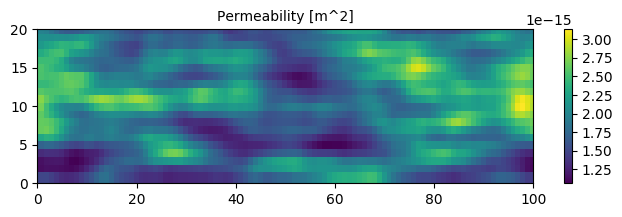### Initial conditions¶

In :
theta_init = createCellVariable(m, theta_res, BCt)
theta_val  = createCellVariable(m, theta_res, BCt)
p_init     = createCellVariable(m, p_res, BCp)
p_val      = createCellVariable(m, p_res, BCp)
rho_init   = createCellVariable(m,
polyval(rho_fit, theta_init.value+T0))
rho_val    = copyCell(rho_init)
mu_init    = createCellVariable(m,
polyval(mu_fit, theta_init.value+T0))
mu_val     = copyCell(mu_init);


### Solver settings and discretization¶

In :
dt         = (L/Nx)/(u_inj/poros)   # [s] time step
final_time = Nx*dt                  # [s]

# discretization
M_BCp, RHS_BCp = boundaryConditionTerm(BCp)
M_BCt, RHS_BCt = boundaryConditionTerm(BCt)
M_conductivity = diffusionTerm(-harmonicMean(
createCellVariable(m, λ_eff)));


### Solver loop¶

In :
for t in dt:dt:final_time

for i in 1:3 # internal loop
# solve pressure equation
RHS_ddt_p   = constantSourceTerm(poros/dt*(rho_val - rho_init))
water_mobil = harmonicMean(perm_field./mu_val)
rho_face = arithmeticMean(rho_val)
M_diff_p    = diffusionTerm(-rho_face.*water_mobil)
p_val       = solveLinearPDE(m, M_diff_p + M_BCp,
RHS_BCp - RHS_ddt_p)

# velocity vector

# solve heat equation
α                  = poros*rho_val*cp_water+(1-poros)*
rho_rock*cp_rock
M_trans, RHS_trans = transientTerm(theta_init, dt, α)
M_conv             = convectionTerm(cp_water*rho_face.*u)
theta_val          = solveLinearPDE(m,
M_BCt + M_conv + M_trans + M_conductivity,
RHS_BCt + RHS_trans)

# update density and viscosity values
rho_val.value[:] = polyval(rho_fit, theta_val.value + T0)
mu_val.value[:]  = polyval(mu_fit, theta_val.value + T0)
end # end of inner loop

rho_init   = copyCell(rho_val)
p_init     = copyCell(p_val) # not necessary
theta_init = copyCell(theta_val)
end

In :
figure(figsize=(8,2))
visualizeCells(theta_val+T0)
title("temperature profile")
colorbar();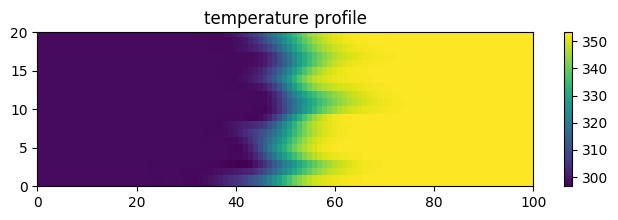Does not look bad at all. Let me plot the temperature profiles in each row of the grid cells, on a 2D plot:

In :
plot(theta_val.value[2:end-1,2:end-1]+T0);
xlabel("Domain length")
ylabel("T [K]")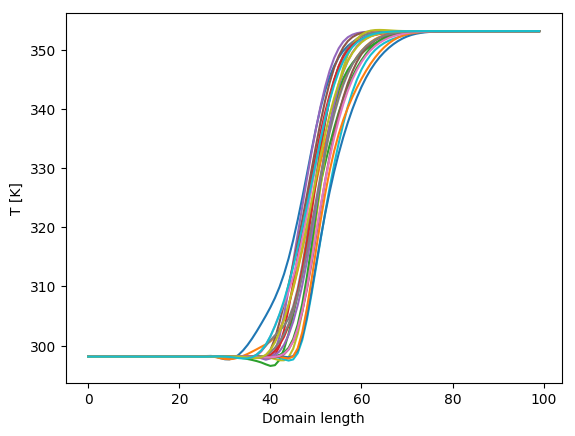Out:
PyObject <matplotlib.text.Text object at 0x7f86465741d0>

You can see that the solution is slightly unstable (temperature goes below the injection temperature, which is impossible according to our model). This can be solved by using the upwind scheme for the convection term in the heat equation.

# Lid-driven flow in a cavity: Navier-Stokes in FVTool

This is a very short post. Kai implemented the lid-driven cavity problem in FVTool, something that I couldn't have done soon or perhaps ever. You can find his implementation here. This is a contour plot of the velocity profile: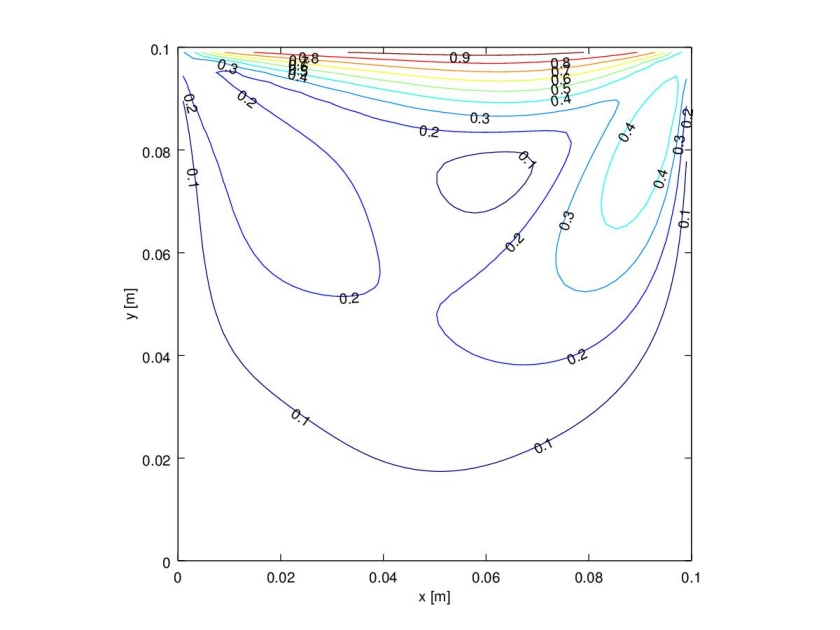In case you look into the files, please don't compare his programming style with mine. My style is quite embarrassing!